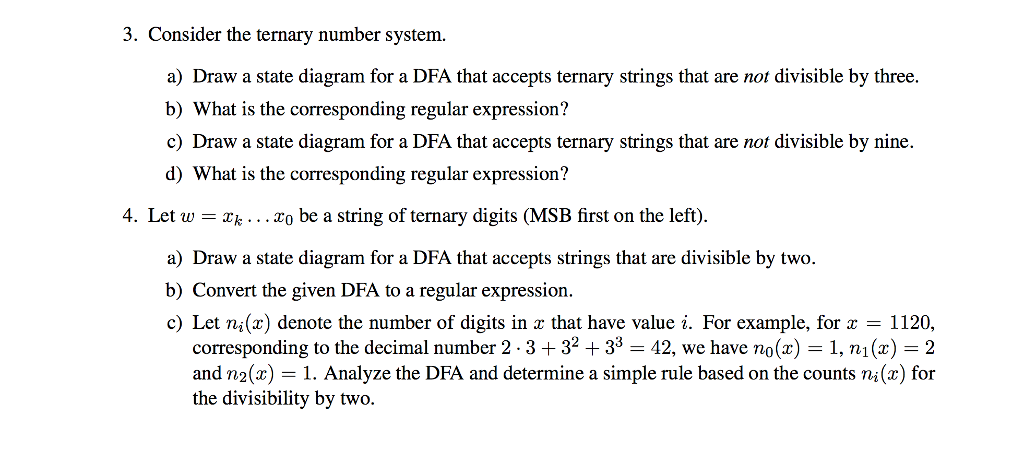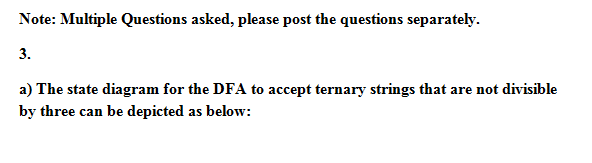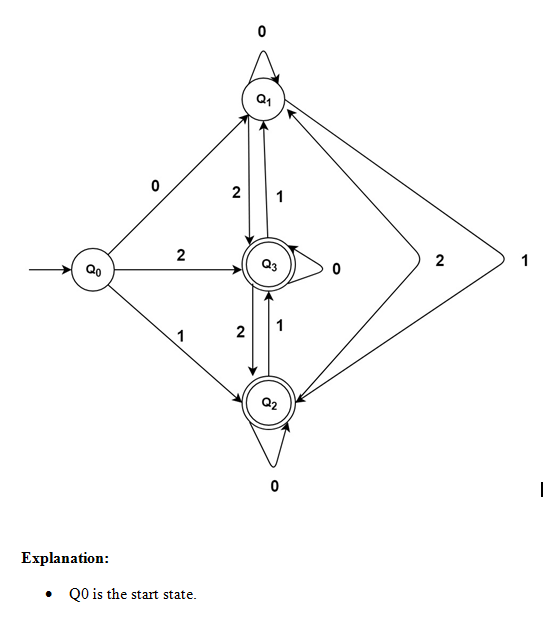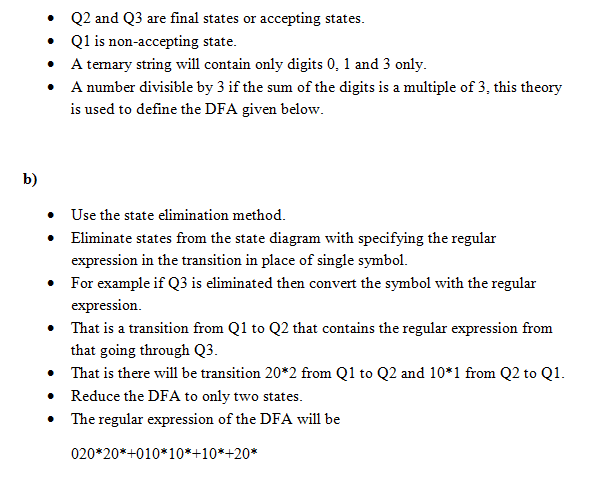# Homework Solution: Consider the ternary number system. a) Draw a state diagram for a DFA that accepts ternary strings th…Consider the ternary number system. a) Draw a state diagram for a DFA that accepts ternary strings that are not divisible by three. b) What is the corresponding regular expression? c) Draw a state diagram for a DFA that accepts ternary strings that are not divisible by nine. d) What is the corresponding regular expression? Let omega = x_k... x_0 be a string of ternary digits (MSB first on the left). a) Draw a state diagram for a DFA that accepts strings that are divisible by two. b) Convert the given DFA to a regular expression. c) Let n_i(x) denote the number of digits in x that have value i. For example, for x = 1120, corresponding to the decimal number 2 middot 3 + 3^2 + 3^3 = 42, we have n_0(x) = 1, n_1(x) = 2 and n_2(x) = 1. Analyze the DFA and determine a simple rule based on the counts n_i (x) for the divisibility by two.